### Test: SAT II Math I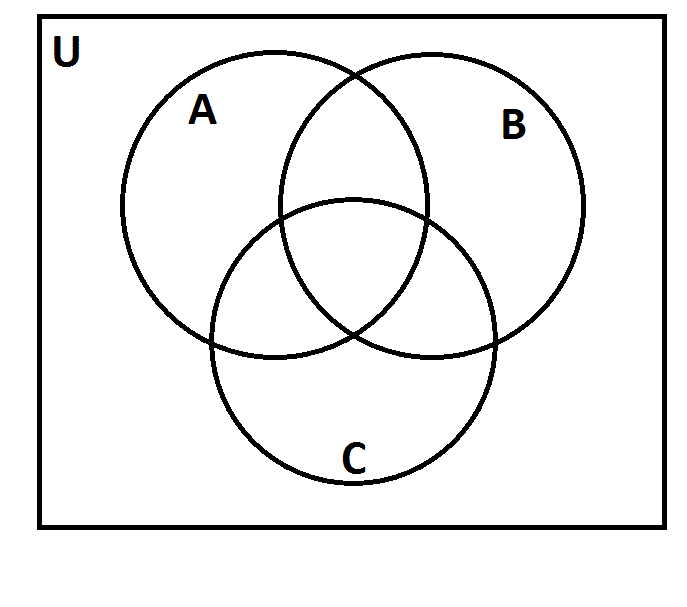1 The above represents a Venn diagram. The universal set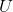is the set of all positive integers. Letbe the set of all multiples of 2; letbe the set of all multiples of 3; letbe the set of all multiples of 5. As you can see, the three sets divide the universal set into eight regions. Suppose each positive integer was placed in the correct region. Which of the following numbers would be in the same region as 450?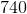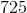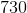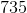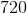1/1 questions

0%

## Access results and powerful study features!

Take 15 seconds to create an account.